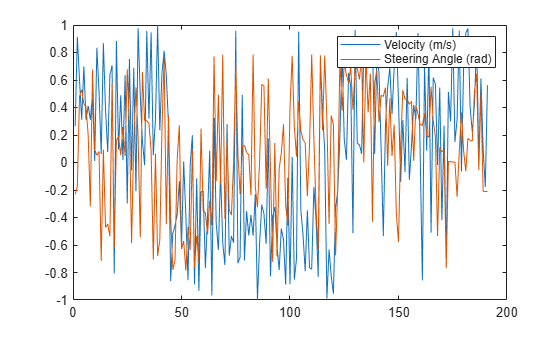# navPathControl

Path representing control-based kinematic trajectory

## Description

The `navPathControl` object stores paths that are typically created by control-based path planners like the `plannerControlRRT` object. The `navPathControl` object represents paths as a sequence of states, controls, durations, and targets. This object associates each path with a specific state propagator, which propagates the control commands to determine the resulting states.

This object specifies states and targets in the path in the state space of propagator. Controls are outputs from a controller that are used to update your systems state during propagation. This object applies each control for an associated duration. Controls can be reference signals or direct inputs to an integrator depending on your system design.

## Creation

### Syntax

``pathObj = navPathControl(propagator)``
``pathObj = navPathControl(propagator,states,controls,targets,durations)``

### Description

example

````pathObj = navPathControl(propagator)` creates a path object with the specified state propagator. The `propagator` argument specifies the `StatePropagator````
````pathObj = navPathControl(propagator,states,controls,targets,durations)` initializes the path with a sequence of specified states, controls, targets, and durations. The input states must have one more row than the other input vectors and matrices.```

## Properties

expand all

State propagator, specified as an object of a subclass of `nav.StatePropagator`. For example, the `mobileRobotPropagator` object represents the state space and kinematic control behavior for different mobile robot vehicle models.

Data Types: `double`

Series of states for the path, specified as an n-by-m matrix. n is the number of points in the path. m is the dimension of the state vector.

You can specify this property at object creation by using the `states` argument.

Data Types: `double`

Control input for each state, specified as an (n–1)-by-m matrix. n is the number of points in the path. m is the dimension of the state vector.

You can specify this property at object creation by using the `controls` argument.

Data Types: `double`

Target state for each state in the path, specified as an (n–1)-by-m matrix. n is the number of points in the path. m is the dimension of the state vector.

You can specify this property at object creation by using the `targets` argument.

Data Types: `double`

Duration of each control input, specified as an (n–1)-element vector in seconds. n is the number of points in the path.

You can specify this property at object creation by using the `durations` argument.

Data Types: `double`

Number of states in the path, specified as a positive integer.

Data Types: `double`

Number of segments between states in the path, specified as a positive integer, which must be one less than the number of states.

Data Types: `double`

## Object Functions

 `append` Add states to end of path `interpolate` Interpolate path based on propagator step size `pathDuration` Total elapsed duration of control path

## Examples

collapse all

Plan control paths for a bicycle kinematic model with the `mobileRobotPropagator` object. Specify a map for the environment, set state bounds, and define a start and goal location. Plan a path using the control-based RRT algorithm, which uses a state propagator for planning motion and the required control commands.

Set State and State Propagator Parameters

Load a ternary map matrix and create an `occupancyMap` object. Create the state propagator using the map. By default, the state propagator uses a bicycle kinematic model.

```load('exampleMaps','ternaryMap') map = occupancyMap(ternaryMap,10); propagator = mobileRobotPropagator(Environment=map); % Bicycle model```

Set the state bounds on the state space based on the map world limits.

```propagator.StateSpace.StateBounds(1:2,:) = ... [map.XWorldLimits; map.YWorldLimits];```

Plan Path

Create the path planner from the state propagator.

`planner = plannerControlRRT(propagator);`

Specify the start and goal states.

```start = [10 15 0]; goal = [40 30 0];```

Plan a path between the states. For repeatable results, reset the random number generator before planning. The `plan` function outputs a `navPathControl` object, which contains the states, control commands, and durations.

```rng("default") path = plan(planner,start,goal)```
```path = navPathControl with properties: StatePropagator: [1x1 mobileRobotPropagator] States: [192x3 double] Controls: [191x2 double] Durations: [191x1 double] TargetStates: [191x3 double] NumStates: 192 NumSegments: 191 ```

Visualize Results

Visualize the map and plot the path states.

```show(map) hold on plot(start(1),start(2),"rx") plot(goal(1),goal(2),"go") plot(path.States(:,1),path.States(:,2),"b") hold off```Display the `[v psi]` control inputs of forward velocity and steering angle.

```plot(path.Controls) ylim([-1 1]) legend(["Velocity (m/s)","Steering Angle (rad)"])```